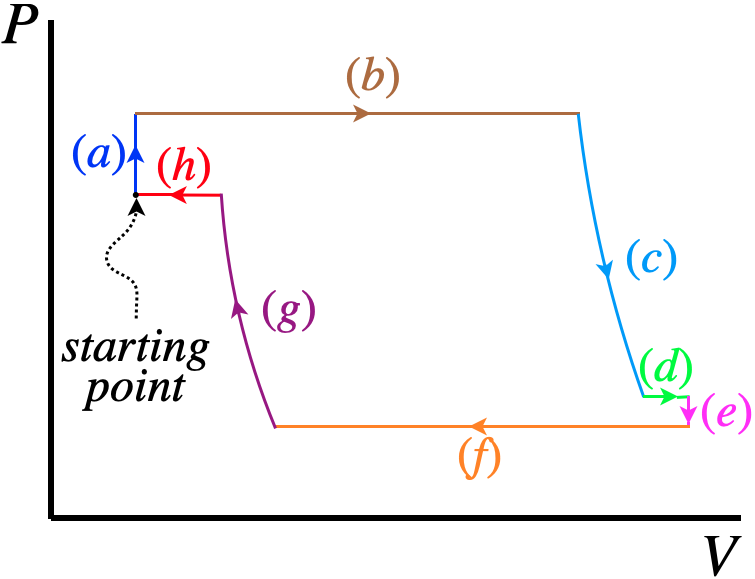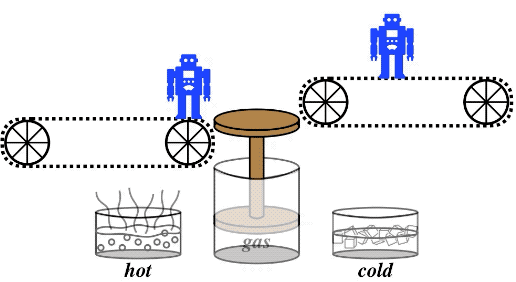$$\require{cancel}$$

# 9.1: Background Material

## Our Heat Engine

In this lab, we will be using a simple heat engine which consists of an airtight piston and two thermal reservoirs. The cold reservoir is an ice water bath at $$273K$$, and the other is boiling water at $$\approx 373K$$. We will extract work from this engine by placing a weight on the piston when the trapped air is cold, then heat the gas so that it expands and the piston raises the weight. After it is raised, the weight is removed, and the engine is returned to its initial state to complete the cycle.

There are several processes involved in this cycle, and a few of these processes are somewhat hidden. Let's look in detail at each one in turn. The approximate shape of the $$PV$$ diagram of the cycle will look something like this:

Figure 9.1.1 – Our Engine CycleNow this cycle looks like quite a mess – certainly there is more going on here than in simple cycles we have discussed in class. But a careful accounting of what is going on physically at each step will make clear the origin of all these little nooks, and the similarity with what we see in our data will be striking.

Starting point: Weight is on piston, and trapped air is at the temperature of the cold reservoir: $$273K$$.

The first thing we are going to do is to switch reservoirs from cold to hot, meaning we will add heat to the trapped air. But immediately we run into a complication. The piston is certainly not frictionless, so it is not unreasonable to assume that at the beginning static friction is assisting the gas in holding up the piston. A free-body diagram that respresents this  is below.

Figure 9.1.2 – Free-Body Diagram of Piston at Starting PointSo what will happen when we heat the gas? Well, the pressure will start to rise increasing the upward force by the gas. But until this increased upward force takes on the duty of the static friction force, and then overcomes the static friction that emerges in the opposite direction, the piston will not start to rise. With the pressure (and temperature) rising while the piston remains in place, we have:

($$a$$) is a short isochoric process

Once the piston finally starts moving, the expansion occurs at a fixed pressure (which is determined by the weight and the kinetic friction), so:

($$b$$) is an isobaric process

Now with the job of lifting the weight complete, it is removed from the piston. This occurs very suddenly, not giving the gas sufficient time to exchange heat with the hot reservoir. With no heat exchanged, we have:

($$c$$) is an adiabatic process

With the adiabatic process complete, we know that the sudden expansion will have cooled the gas to a temperature below where it started, which was the temperature of the hot reservoir. But the engine remains in contact with the hot reservoir for awhile after this process is complete, so naturally the gas will warm-up again. As this occurs, no weight is added or taken away from the piston, so the pressure remains fixed. We therefore expect to see the volume increase slightly due to this small amount of heating of the gas at constant pressure, giving:

($$d$$) is a short isobaric process

Once the piston has come to rest at its peak height, it is time to cool the trapped air back to its starting temperature, so we once again bring it into contact with the cold reservoir. The weight and pressure are both considerably smaller than before, so the static friction force present should also be quite small (and really it could be pointing in either direction). Its effect will be little more than noise in this experiment, but we include it in step (e) for consistency. Once again, while static friction is present, the volume doesn't change, so we get:

($$e$$) is a very short isochoric process

After breaking free of the static friction, the cooling of the trapped air once again occurs at constant pressure:

($$f$$) is an isobaric process

In order to complete the cycle, we have to return the engine precisely back to where it started. The colder reservoir is now in place, but unlike before, there is no weight on the piston, so we return that. This we do suddenly, which like before means that there is no time for heat exchange, giving:

($$g$$) is an adiabatic process

And finally, as we saw after the previous adiabatic process, the temperature has to equalize with the reservoir. This time, the sudden compression resulted in the trapped air getting hotter than the cold reservoir, which means that it cools and contracts in volume at constant pressure to equalize:

($$h$$) is a short isobaric process

After looking so closely at all these details, it is useful to step back and see what the end result is. We started and ended with a weight at a low elevation. Along the way, we lifted a weight to a higher elevation and removed it. We can imagine doing this over-and-over, lifting a long line of weights coming in on a conveyer belt to higher levels:

Figure 9.1.3 – The Net Result of Our EngineAs you can see, for every cycle, some heat enters the engine, less heat leaves it, and the balance of the energy goes into raising the potential energy of the weight. The work doesn't go back into the gas upon compression, because the weight is removed after it is lifted.

[As you will see in the videos, the way that each reservoir is activated is by moving a cannister from ice water to boiling water, and back.  The cannister shares air with the interior of the piston through a rubber tube that connects the two bodies of air, forming a single sealed container of continuous gas.]

## Engine Efficiency

The efficiency of this (and any) engine is the ratio of the net work that comes out and the heat that comes in from the hot reservoir (the heat that exits into the cold reservoir is not included). This engine is connected to the hot reservoir during legs ($$a$$) though ($$d$$). We know what types of processes these are, so with this information and the fact that air is a diatomic molecule, we can determine the heat transferred during each process in terms of changes of pressure and/or volume that we read off the PV diagram.

When you make this calculation in the lab that follows, you may be troubled by how low the efficiency comes out to be. To assuage your fears, you may want to consider that if this was the most efficient engine possible (a Carnot engine, which follows only isothermal and adiabatic curves), for the two temperatures we have available, the efficiency would be:

$e = 1-\dfrac{T_C}{T_H} = 1-\dfrac{273K}{373K} = 27\%$

Now when you take into account that we are assuming that the various processes are reversible (which they clearly are not, since the temperature difference between the gas and the reservoir is virtually always finite), we should expect the efficiency to be significantly lower than this number.